# 第一次作业

PTA第一次作业作业

1.设计思路
（1）算法

（2)流程图：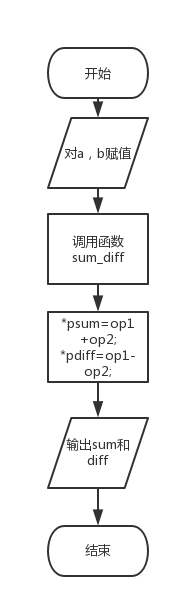2.实验代码：void sum_diff( float op1, float op2, float *psum, float *pdiff ){
*psum=op1+op2;
*pdiff=op1-op2;
}

3.本题调试过程碰到问题及解决的办法：

错误原因：在函数定义时在括号后加一个";"

改正方法：删除";"；

1.设计思路

（1）算法：

（2）流程图：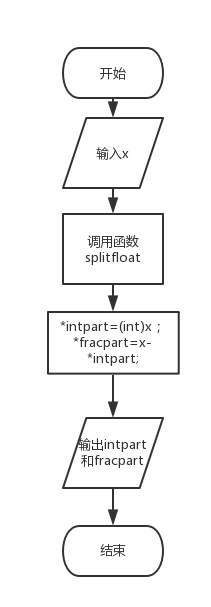2.实验代码：

void splitfloat( float x, int *intpart, float *fracpart ){
*intpart=(int)x;
*fracpart=x-*intpart;
}

3.本题调试过程碰到问题及解决的办法：

错误原因：强制转化的格式错误，错误：int（x）；

改正方法：int放在括号内，加上强制转化的数，正确格式：（int）x；

#### 6-1 在数组中查找指定元素

1.设计思路

（1）算法：

（2）流程图：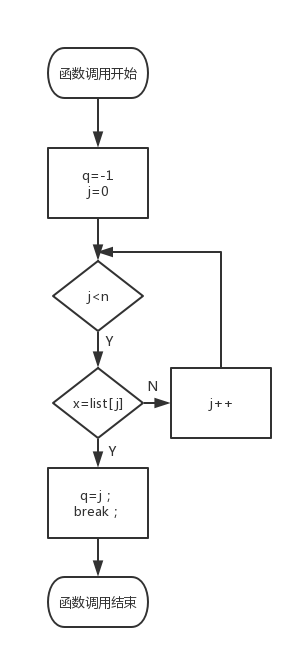2.实验代码：

    int search( int list[], int n, int x ){
int j,q=-1;
for(j=0;j<n;j++){
if(x==list[j]){
q=j;
break;
}
}
return(q);
}

3.本题调试过程碰到问题及解决办法：

#### 6-2 找最大值及其下标

1.设计思路

（1）算法：

（2）流程图：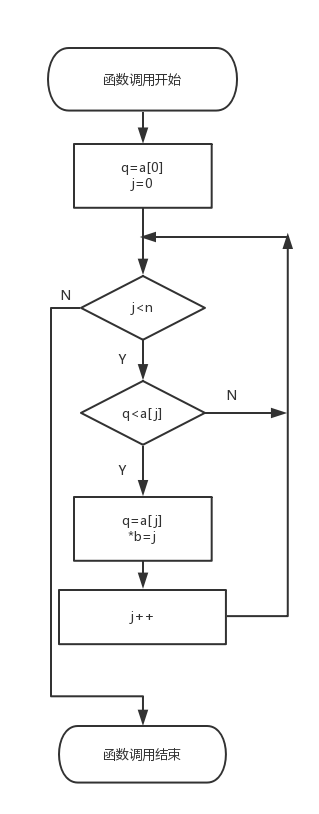2.实验代码：

    int fun(int *a,int *b,int n){
int q=a;
int j;
for(j=0;j<n;j++){
if(q<a[j]){
q=a[j];
*b=j;
}
}
return(q);
}

3.本题调试过程碰到问题及解决办法：

C高级PTA作业（3）

#### 6-1 最小数放前最大数放后

1.设计思路：

（1）算法：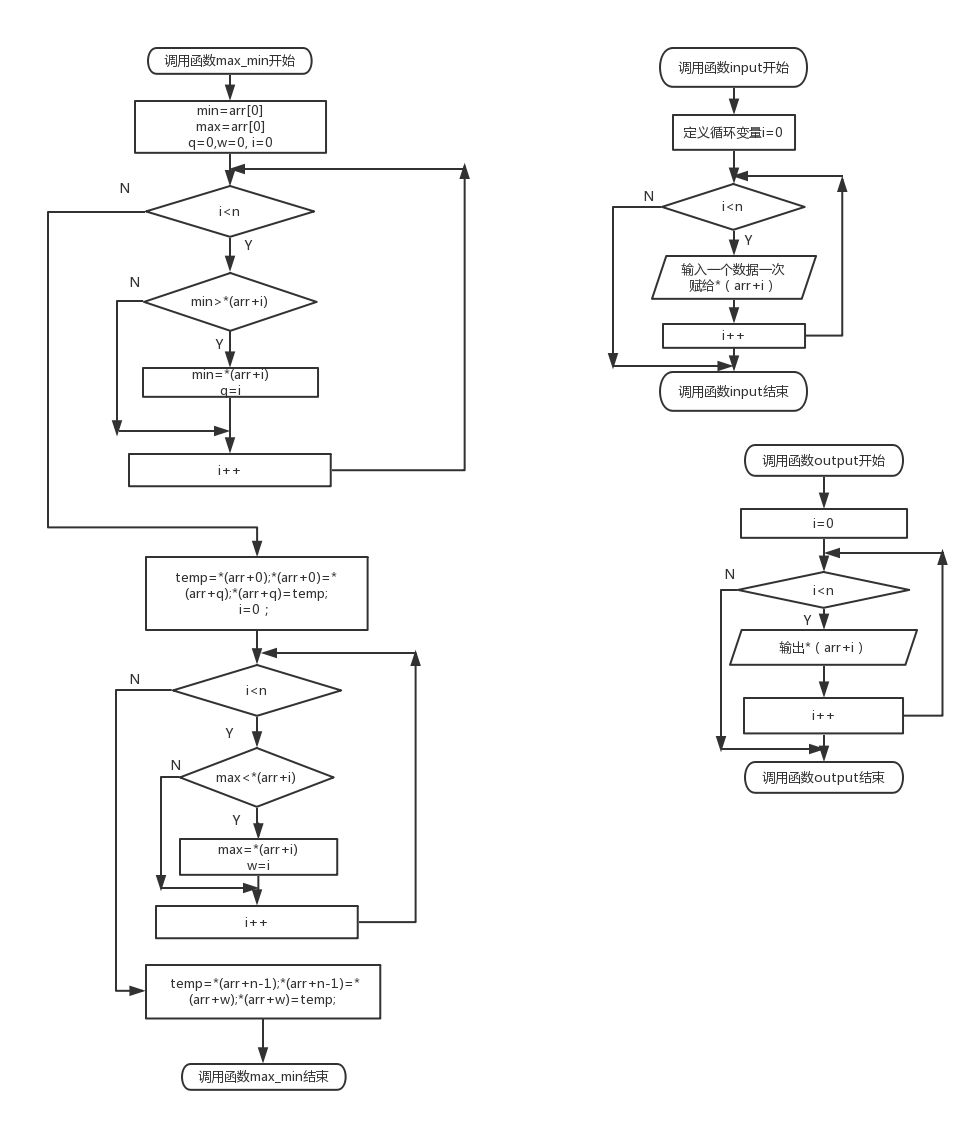2.实验代码：

void input(int *arr,int n){
int i;
for(i=0;i<n;i++){
scanf("%d",arr+i);
}
}
void max_min(int *arr,int n){
int min=arr,max=arr;
int i,temp;
int q=0,w=0;
for(i=0;i<n;i++){
if(min>*(arr+i)){
min=*(arr+i);
q=i;
}
}
temp=*(arr+0);*(arr+0)=*(arr+q);*(arr+q)=temp;
for(i=0;i<n;i++){
if(max<*(arr+i)){
max=*(arr+i);
w=i;
}
}
temp=*(arr+n-1);*(arr+n-1)=*(arr+w);*(arr+w)=temp;
}
void output(int *arr,int n){
int i;
for(i=0;i<n;i++){
printf("%3d",*(arr+i));
}
}

3.本题调试过程碰到问题及解决办法：

#### 6-2 指针选择法排序

1.设计思路：

（1）算法：

（2）流程图：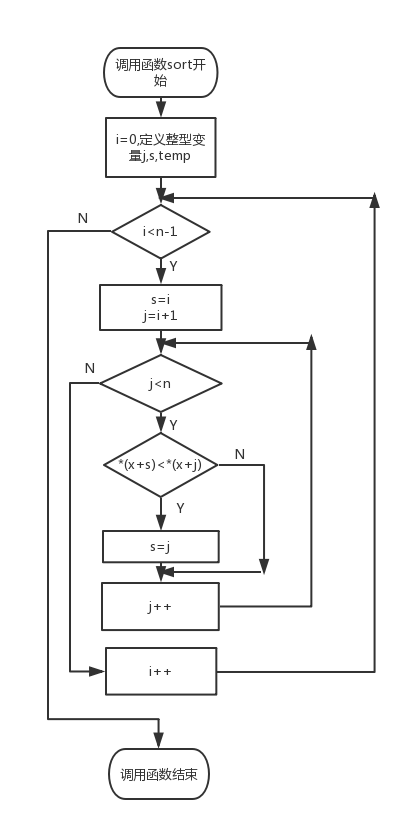2.实验代码：

void sort(int *x,int n){
int i,j,s,temp;
for(i=0;i<n-1;i++){
s=i;
for(j=i+1;j<n;j++){
if(*(x+s)<*(x+j)){
s=j;
}
}
if(s!=i){
temp=*(x+i);*(x+i)=*(x+s);*(x+s)=temp;
}
}
}

3.本题调试过程碰到问题及解决办法：

#### 6-1 判断回文字符串

1.设计思路：

（）算法：

（2）.流程图2.实验代码：

    bool palindrome( char *s ){
int i,count=0,q;
count=strlen(s);
q=count/2;
for(i=0;i<q;i++){
if((*(s+i))!=(*(s+count-i-1))){
return(false);
}
}
if(i==q){
return(true);
}
}

3.本题调试过程碰到问题及解决办法

#### 6-2 使用函数实现字符串部分复制

1.设计思路：

（1）算法：

（2）流程图：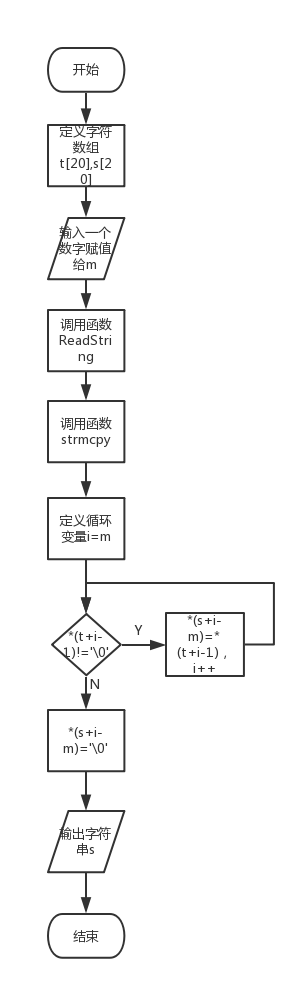2.实验代码：

    void strmcpy( char *t, int m, char *s ){
int i;
for(i=m;*(t+i-1)!='\0';i++){
*(s+i-m)=*(t+i-1);
}
*(s+i-m)='\0';
}

3.本题调试过程碰到问题及解决办法：

我评论谁的作业：

1.进度表：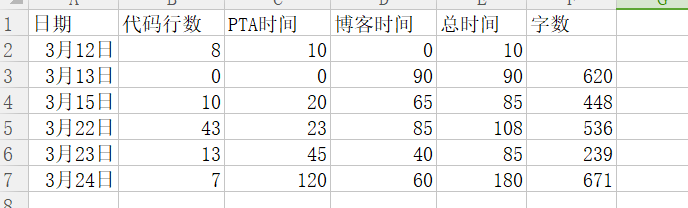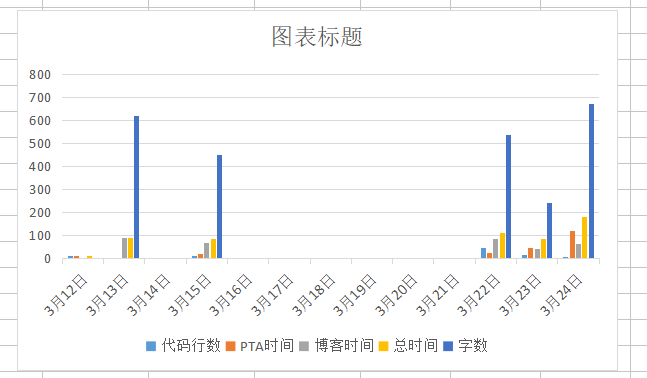2.两周来所有PTA作业中每个题目所使用的知识点。

strlen函数是计算字符数组的实际长度，回文序列判断条件相等字符对应的下标和是数组元素个数-1，

3.我的收获：

%u是输出无符号数所用的格式符，逗号表达式的求解过程：先求表达式1.再求表达式2，逗号表达式的值是表达式是2的值，逗号运算符优先级时最低的，%md是输出的整数占三列

&：取地址运算符，*：指针运算符或间接访问运算符

int *p=a将数组首元素的地址赋给指针变量p，

*p++先算*p再算++，*（p+i）=a[i];输出字符串时用%s，

posted @ 2018-03-13 21:04  _晨曦  阅读(347)  评论(26编辑  收藏  举报